SSAT Upper Level Math : Parallel Lines

Example Questions

1 2 3 4 6 Next →

Example Question #182 : Lines

Find the equation of the line that passes through the point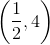and is parallel to the line with the equation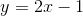.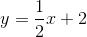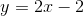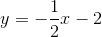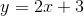Explanation:

Because the two lines are parallel, we know that the slope of the line we need to find must also be.

Next, plug in the point given by the question to find the y-intercept of the line.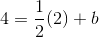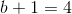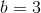Now, we know the equation of the line must be.

Example Question #21 : How To Find The Equation Of A Parallel Line

Find the equation of the line that passes through the point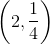and is parallel to the line with the equation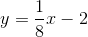.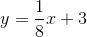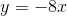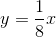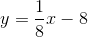Explanation:

Because the two lines are parallel, we know that the slope of the line we need to find must also be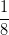.

Next, plug in the point given by the question to find the y-intercept of the line.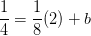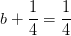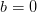.

We can then write the equation of the line: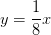Example Question #7 : How To Find The Equation Of A Parallel Line

Which of these formulas could be a formula for a line perpendicular to the line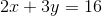?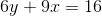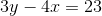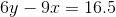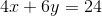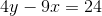Explanation:

This is a two-step problem. First, the slope of the original line needs to be found. The slope will be represented by "" when the line is in-intercept form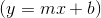.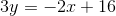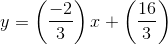So the slope of the original line is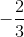. A line with perpendicular slope will have a slope that is the inverse reciprocal of the original. So in this case, the slope would be. The second step is finding which line will give you that slope. For the correct answer, we find the following: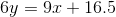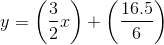So, the slope is, and this line is perpendicular to the original.

Example Question #51 : Parallel Lines

Find the equation of a line that goes through the point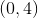and is parallel to the line with the equation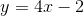.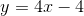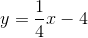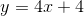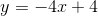For lines to be parallel, they must have the same slope. The slope of the line we are looking for then must be.
Using these two pieces of information, we know that the equation for the line must be